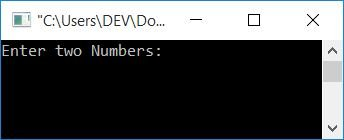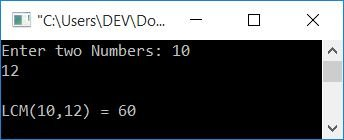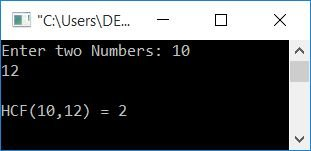# C Program to Find the LCM and HCF of Two Numbers

In this article, you will learn and get code for finding the LCM and HCF of any two given numbers by the user (at run-time). But before going through the program, if you are not aware of how to find LCM and HCF, then I recommend that you go through the following two articles:

I'm sure, within a minute, you will get a complete understanding of both topics. Let's move on and implement it in a C program.

## Find the LCM of two numbers in C

Let's first create a program to find out the LCM of two numbers:

```#include<stdio.h>
#include<conio.h>
int main()
{
int num1, num2, mp;
printf("Enter two Numbers: ");
scanf("%d %d", &num1, &num2);
if(num1>num2)
mp=num1;
else
mp=num2;
while(1)
{
if((mp%num1==0) && (mp%num2==0))
break;
else
mp++;
}
printf("\nLCM(%d,%d) = %d", num1, num2, mp);
getch();
return 0;
}```

This program was built and runs in the Code::Blocks IDE. Here is its sample run:Now supply any two numbers as input, say 10 and 12. Then press the ENTER key to see the following output:Because LCM is the number that must be divisible by both numbers (with a 0 remainder), For example, if 10 and 12 are the two numbers, To find its LCM, we have to first check which one is greater. Initialize the greater number to a variable, say mp. Now using the statement:

```if((mp%num1==0) && (mp%num2==0))
break;```

We have checked whether the value in mp is divisible by both numbers or not. If it is, then exit the while loop using a break statement. Otherwise, increment its value and continue for the next check. On exiting the loop, we have a value stored inside mp that will be equal to the LCM of two given numbers. When you use 1 as the condition in a while loop, it will run indefinitely until the break statement is executed.

There are multiple approaches you can take to calculate LCM or HCF. It is up to you.

### Find LCM in C without using if-else

Now let's create the same-purpose program without using if-else as given below:

```#include<stdio.h>
#include<conio.h>
int main()
{
int num1, num2, lcm, a, b, temp;
printf("Enter two Numbers: ");
scanf("%d %d", &num1, &num2);
a=num1;
b=num2;
while(b!=0)
{
temp = b;
b = a%b;
a = temp;
}
lcm = (num1*num2)/a;
printf("\nLCM(%d,%d) = %d", num1, num2, lcm);
getch();
return 0;
}```

This will produce the same output as the previous one.

## Find the HCF of two numbers in C

Now let's create a program that finds the HCF of two numbers entered by the user:

```#include<stdio.h>
#include<conio.h>
int main()
{
int num1, num2, mp;
printf("Enter two Numbers: ");
scanf("%d %d", &num1, &num2);
if(num1>num2)
mp=num1;
else
mp=num2;
while(1)
{
if((num1%mp==0) && (num2%mp==0))
break;
else
mp--;
}
printf("\nHCF(%d,%d) = %d", num1, num2, mp);
getch();
return 0;
}```

Here is its sample run:As you can see from the above program, we have just reversed the condition of "if" block from:

`(mp%num1==0) && (mp%num2==0)`

to

`(num1%mp==0) && (num2%mp==0)`

And the statement from

`mp++;`

to

`mp--;`

If you have visited both articles (How to Find LCM and HCF) as mentioned at the beginning of the program, then you have a complete understanding of the reversal of the two, that is, the condition and the statement.

Because in HCF we have to find the highest common factor of both numbers, we have initialized the greater number to mp and checked whether it is divisible by both numbers or not. If it is, then break out of the loop; otherwise, decrement its value and continue to check for the next value. For example, if the two given numbers are 18 and 27, the program flow goes like this:

• The highest number, 27, gets initialized to mp.
• The number 1 as a condition of the while loop always evaluates to true.
• As a result, program flow continues until the break keyword is encountered.
• Inside the loop, using an if block, it is checked whether both the numbers, 18 and 27, are divisible by 27 or not.
• Because it is not divisible by the first number 18, otherwise the statement in the block is executed and the value of mp is decremented.
• When the value of mp becomes 9.
• Then, in the if block's condition, because both 18 and 27 are completely divisible by 9.
• As a result, program flow is directed inside the if block's statement, and break; is executed. This exits the loop.
• And finally, print the value of mp as the HCF result of two given numbers.

### Find HCF in C using the while loop

Now let's create another program to find out the HCF of two numbers without using an if-else block.

```#include<stdio.h>
#include<conio.h>
int main()
{
int num1, num2, a, b, temp, hcf;
printf("Enter two Numbers: ");
scanf("%d %d", &num1, &num2);
a=num1;
b=num2;
while(b!=0)
{
temp = b;
b = a%b;
a = temp;
}
hcf = a;
printf("\nHCF(%d,%d) = %d", num1, num2, hcf);
getch();
return 0;
}```

#### The same program in different languages

C Quiz

« Previous Program Next Program »